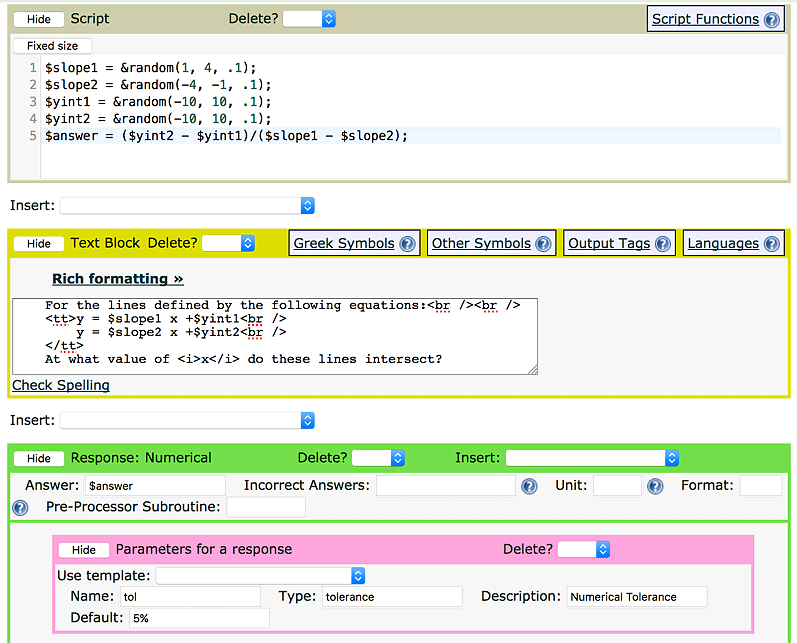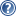###LON-CAPA HelpFigure 1: Slope Problem Parameters

Now you have all the tools to create those wonderful dynamic, randomized problems that you've seen in LON-CAPA. Consider a Numerical Response problem where the equations for two lines are randomly generated and the students are asked to find the intercept. Try filling out your problem with the parameters shown in Figure.

When creating randomized problems, you want to make sure that the problems always have an answer. Consider what might happen if two slopes are chosen, both with the expression &random(-1.0,1.0,.2). One out of ten students would get a problem where both slopes were equal, which has either no solution (for unequal y-intercepts) or an infinite number of solutions (for equal slopes and y-intercepts). Both of these cause a division-by-zero error on the division that computes the answer. There are many ways to avoid this, one of the easiest of which is picking one slope negative and one positive. This same problem can show up in many other places as well, so be careful.